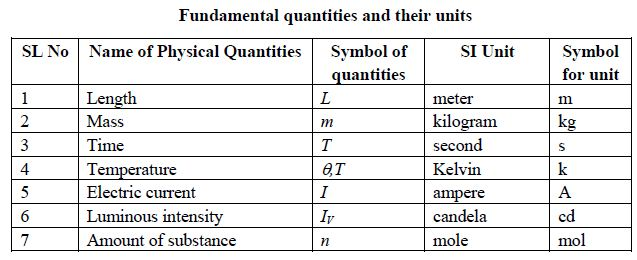# What are Fundamental Unit in SI?

We can select fundamental units according to your liking, since the units of fimdamental quantities do not depend on other units. But our selection must have international recognition. It should have some characteristics as well. For example, it should be unchangeable, that is, independent of place, time and person. It will not change due to passage of time or any other natural change. It could be reproduced easily.

The standard of fundamental units that were accepted in 1960, while introducing the SI system of units, were changed later on in some cases in order to attain suitable characteristics. But no change was brought in values of the units. For example, at present, meter is defined in terms of distance traveled by light. Earlier, meter was defined using wavelength of a kind of light. Prior to it, the length of a and kept at Sevres near Paris in France was taken as the standard of meter. The latest accepted standard of fundamental units in International System are described below:

Unit of length : Meter : The distance traveled by light in vacuum (air-free space) in (1/ 299792458) second is defined as one meter (n).

Unit of mass : Kilogram : The kilogram is the mass equal to that of a cylinder made of platinum-iridium alloy (International prototype kilogram) kept at the International Bureau of Weights and Measures at Severs, France. The diameter of this cylinder is 3.9 cm ; its height is also 3.9 cm.

Unit of time : Second :The time required to complete 9 192 631 770 vibrations by a caesium-133 atom is called one second (s).

Unit of temperature : Kelvin : The temperature which equals to (1/273.16) of the thermodynamic temperature of the triple point of water is called one Kelvin (k).

Unit of electric current : Ampere : The ampere is that current which produces a force of 2 x 10-7 Newton per meter in vacuum between two parallel infinitely long conductors of negligible cross-sectional area 1 meter apart when each conductor carries the same current.

Unit of luminous intensity: Candela : Candela is the quantity of luminous intensity of any source of light which radiates monochromatic radiation at a particular direction with 1 a frequency of 540 * 1012 Hz and emissive power of 1/683 watt per steredian solid angle.

Unit of amount of substance : Mole : The mole is defined as the amount of substance which contains elementary entities (e.g atoms, molecules, ions, electrons etc. or any specified group of these particles) equal to the number of atoms in 0.012 kilogram of Carbon-12.